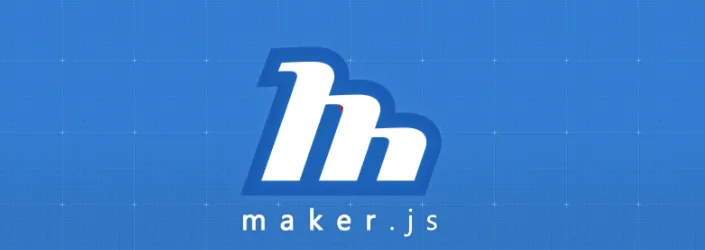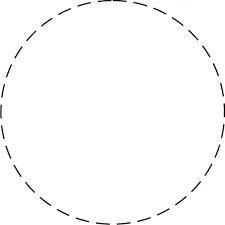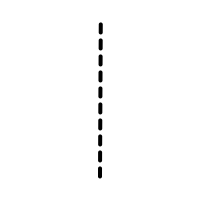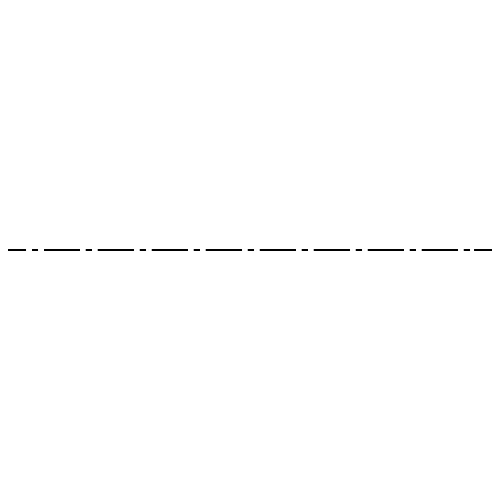# Maker.Js for CNC and laser cutters###### Published:Tue Sep 05 2023##### Summary

By using Maker.js you can draw using geometry and drafting. Initially designated for CNC and laser cutters, Maker.js can also help you programmatically draw shapes for any purpose. It runs on both Node.js and web browsers.

Core Features of Maker.js

• paths — The primitive elements of a drawing are lines, arcs, and circles.
• models — Groups of paths to compose a shape.
• layers — Organization of models, such as by color or tool type.
• chains — A series of lines and arcs that connect end-to-end continuously.

In Node.js or React you can install Maker.js by using this code.

```npm install makerjs --save
// Include it on our program.
var makerjs = require('makerjs');```

In Paths, you can draw a line, circle, or arc by using these codes which require us to pass some default parameters. For example, when drawing a line, there's a start-point and end-point; for drawing a circle, there must be a radius or a diameter and an origin of the circle. Similarly, while drawing an arc, there is a start-point and end-point of an arc, radius. The arc is clockwise and the large arc is anti-clockwise.

Let's see the code for that.

For drawing a line,

```var line = {
type: 'line',
origin: [0, 0],
end: [1, 1]
};```

For drawing a circle,

```var circle = {
type: 'circle',
origin: [0, 0],
};
```

For drawing an arc,

```var arc = {
type: 'arc',
origin: [0, 0],
startAngle: 0,
endAngle: 45
};```

For more reference:https://maker.js.org/docs/basic-drawing/#content

We can also draw a dashed circle by using this code.

```const div = 360/50; // 50 is total dashed lines in circle
const span = div * 0.70; // distance b/w dashed line
const paths=[];
for(var i = 1; i < 51;i++){
const start = i * div;
}
return paths;```For a dashed line, you can use the following code.

```const count = 30; //Total count of lines
const ratio = 0.6; //Distance b/w 2 lines
const paths = [];
const line = new makerjs.paths.Line(startPoint,endPoint);
const length = makerjs.measure.pathLength(line);
const totalDashLength = length * ratio;
const totalGapLength = length — totalDashLength;
const dashLength = totalDashLength / count;
const gapLength = totalGapLength / (count — 1);
const normal = makerjs.point.subtract(line.end, line.origin);
const dash = new makerjs.paths.Line([0, 0], makerjs.point.scale(normal, dashLength / length));
const gap = makerjs.point.scale(normal, gapLength / length);
var origin = line.origin;
for (var i = 0; i < count; i++) {
const d = makerjs.\$(dash)
.clone()
.move(origin)
.\$result;
paths.push(d);
}
return paths;```In Models, we can draw a Bolt circle, Star, Bezier Curve, Ellipse, Round Rectangle Etc.

Example of Ring and Boltcircle in models

```var makerjs = require('makerjs');

var model = {
models: {
ring1: new makerjs.models.Ring(40, 100),
bc1: new makerjs.models.BoltCircle(90, 4, 10),
bc2: new makerjs.models.BoltCircle(55, 7, 6, 30)
}
};

var svg = makerjs.exporter.toSVG(model);

document.write(svg);```

For more reference:https://maker.js.org/docs/basic-drawing/#Models

We can also find the intersection point of paths by,

```var makerjs = require('makerjs');

var model = {
paths: {
line1: new makerjs.paths.Line([0, 0], [20, 10]),
line2: new makerjs.paths.Line([2, 10], [50, 2])
}
};

var int = makerjs.path.intersection(model.paths.line1, model.paths.line2);
console.log(int);
var svg = makerjs.exporter.toSVG(model);

document.write(svg);
```

You can also draw a small or big line or circle,

Let's take a look at the code for small and big dashed lines,

```var paths5=[]
var k=-800;
for(var s = 0; s < 800/37; s++){
if(s%2 === 0){
k = k+90
paths.push(new makerjs.paths.Line([k+40,0],[k,0]))
}
else{
k=k+60
paths.push(new makerjs.paths.Line([k+80,0],[k,0]))
}
}
return paths5;```The code for small and big dashed arcs in a circle is,

```const div = 360/30;
const paths = [];
for(var i = 1; i < 31; i++){
if(i % 2 === 1){
const span = div * 0.7;
const start = i * div;
}
else{
const span = div * 0.55;
const start = i * div;
}
}
return paths;```For Exporting.

Maker.js provides SVG, DXF, and OpenCad Format for exporting our canvas drawing.

For SVG,

Callmakerjs.exporter.toSVG(model)to pass your model. This function returns a string of SVG.

For DXF,

Callmakerjs.exporter.toDXF(model)to pass your model. This function returns a string of DXF.

If your drawing has layers with names that match the following reserved color names, paths on that layer will have the following stroke color automatically:
aqua, black, blue, fuchsia, green, gray, lime, maroon, navy, olive, orange, purple, red, silver, teal, white, yellow.

For more reference:https://maker.js.org/docs/exporting/#content

For more documentation:https://maker.js.org/

# Maker.Js for CNC and laser cutters# Are you Struggling with Building your Dream Web or Mobile Application?## Looking for career opportunity ?career@bitontree.comReach us out